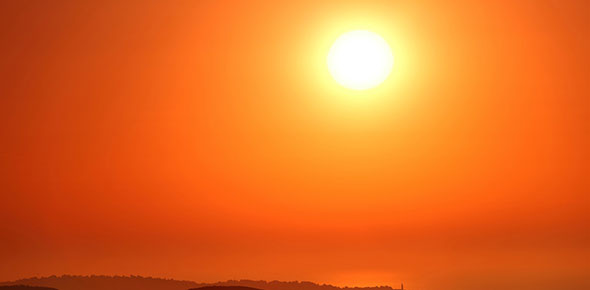# Our Sun Chapter Quiz And Stars Quiz

26 Questions | Total Attempts: 120SettingsThe solar system is the combination of the sun, moon, stars and planets. The quiz below tests your knowledge on the sun and the stars. How well do you know these two components of the solar system? Find out below!

• 1.
How hot is the core of our sun?
• A.

14 million

• B.

15,600,000

• C.

13 million

• D.

5 million

• E.

-15

• 2.
How hot is the radiative zone?
• A.

8 million degrees celcius

• B.

8 billion degrees celcius

• C.

6.5 million degrees celcius

• D.

2 million degrees celcius

• E.

2 billion degrees celcius

• 3.
What does E=mc2 predict?
• A.

Mass conversion

• B.

The amount of fusion that is made

• 4.
light has the longest wave length
• A.

Red

• B.

Violet

• 5.
What color has the longest wavelength
• A.

Orange

• B.

Violet

• 6.
is used to seperate starlight into colors
• A.

Telescope

• B.

Starlight separater 2000

• C.

Spectroscope

• 7.
A                      spectrumis an unbroken band of colors, which shows that it's source is emitting light off all visible wavelength's.
• 8.
An               spectrum is a series of unevenly spaced lines of different colors and brightness
• 9.
spectrum is a continuous spectrum crossed by dark lines.
• 10.
What is the dopplor effect?
• 11.
What spectra can be used to determine the composition of a planet's atmoshere?
• A.

Continuous

• B.

Absorption

• C.

Emission

• 12.
What does a spectroscope do?
• A.

• B.

Redshift of a planet

• C.

Blueshift of a planet

• 13.
• A.

In the Form of waves

• B.

Through the visible light section

• 14.
All stars get their energy from what?
• A.

Fision

• B.

Fusion

• C.

Compression

• D.

Plasma

• 15.
How many earth's can fit inside our sun?
• A.

1 billion

• B.

1 trillion

• C.

1 thousand

• D.

1 million

• 16.
When nuclei fuse they create what?
• A.

Plasma

• B.

Energy

• 17.
What is the largest star?
• A.

Epsilon Aurigae

• B.

Goliath

• C.

• 18.
The suns core consists of what?
• A.

Hydrogen

• B.

Helium

• C.

Plasma

• 19.
How far away is the sun from our earth
• A.

100 million

• B.

150 million

• C.

200 million

• D.

751 million

• 20.
Sunspots happen on hich of our planets
• A.

Venus

• B.

Sun

• C.

Earth

• D.

Pluto

• 21.
Electronically charged particles given off by the corona are called what?
• A.

Province wind

• B.

Strong wind

• C.

Aurora

• D.

Solar winds

• 22.
His gas different from plasma?
• A.

Plasma has charged particles

• B.

Gas is heavier

• C.

Plasma is heavier

• 23.
Where does earth get it's energy?
• A.

Nuclear Fusion

• B.

Diesel

• C.

• 24.
Is the frequency of sunspots predictable?
• A.

Yes

• B.

No

• 25.
What year cycle is the frequency of sunspots predictable?
• A.

1 year

• B.

5 years

• C.

6 years

• D.

11 years

Related TopicsBack to top